# SVM - Understanding the math - Duality and Lagrange multipliers

This is the Part 6 of my series of tutorials about the math behind Support Vector Machines.
Today we will learn about duality, optimization problems and Lagrange multipliers.

If you did not read the previous articles, you might want to start the serie at the beginning by reading this article: an overview of Support Vector Machine.

## Duality

In mathematical optimization theory, duality means that optimization problems may be viewed from either of two perspectives, the primal problem or the dual problem (the duality principle). The solution to the dual problem provides a lower bound to the solution of the primal (minimization) problem.  (Wikipedia)

The concept of duality is pretty simple to understand if you know what a lower bound is.

### What is a lower bound?

If you have a partially ordered set $K$ (a set having comparable elements, where the relation "less than or equal" can be used), the lower bound is an element of $K$ which is less than or equal to every element of $S$.

To be less abstract. If you pick a real number (from the partially ordered set $\mathbb{R}$) and it is less than or equal to every element of a subset of $\mathbb{R}$, then you can call this element a lower bound.

Example:

Let us consider the subset of $\mathbb{R}$:

• Because 1 is less than or equal to 2, 4 ,8 and 12, I can say that 1 is a lower bound of S.
• The same is true for -3 for instance.
• And even if it is in S we can also call 2 a lower bound of S.

Moreover, because 2 is larger than any other lower bounds, we can give it a special name, we call it the infimum (or greatest lower bound).

So in our example, you can find an infinity of lower bound, but there is only one infimum.

Note: The same logic apply with the relation "greater than or equal" and we have the concept of upper-bound and supremum.

### Coming back to duality

Now that we know what a lower bound is, what do we understand about the definition of duality? Well, this definition means that if you have a minimization problem, you can also see it as a maximization problem.  And when you find the maximum of this problem, it will be a lower bound to the solution of the minimization problem, i.e. it will always be less than or equal to the minimum of the minimization problem.

## Why do we care about duality?

It turns out that sometimes, solving the dual problem is simpler than solving the primal problem. From what we saw about lower bounds, we can see that for some problems solving the dual problem gives us the same result as solving the primal problem!   But when?

Let us look at a visual illustration.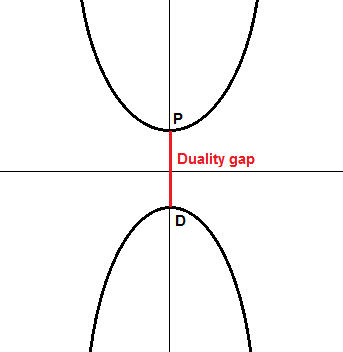In the schema above, imagine that in our primal problem, we are trying to minimize the function at the top of the graph. Its minimum is the point $P$. If we search for a dual function, we could end up with the one at the bottom of the graph, whose maximum is the point $D$. In this case, we clearly see that $D$ is a lower bound. We defined the value $P-D$ and call it the duality gap. In this example, $P-D > 0$ and we say that weak duality holds.

In the schema below, we see that $P-D=0$, there is no duality gap, and we say that strong duality holds.## Optimization problems with constraints

#### Notation

An optimization problem is typically written:

This notation is called the standard form. You should know that there are others notations as well.

In this notation, $f$ is called the objective function (it is also sometimes called the cost function). By changing $x$ (the optimization variable) we wish to find a value $x^*$ for which $f$ is at its minimum.

There is also $p$ functions $g_i$ which define equality constraints and $m$ functions  $h_i$ which define inequality constraints.

The value we find MUST respect these constraints!

### What does it mean to respect the constraints?

Imagine you try to solve the following optimization problem:

There is no constraint, so finding the minimum is easy, the function $x^2$ is 0 when $x = 0$. This is shown with a red star on the graph below: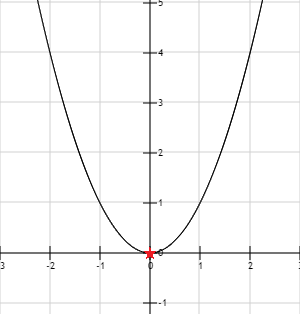When there is no constraint the minimum is zero

#### Equality constraints

However, what if we try to add an equality constraint? For instance, we want to find the minimum, but we must ensure that $x=1$. It means we need to solve this optimization problem:

This time, when we try $x=0$ we see that the function returns its minimum, however, we cannot say this is the solution of our optimization problem. Indeed, the constraint $x=1$ is violated. In this example, our only choice is to use $x=1$ and this is the solution.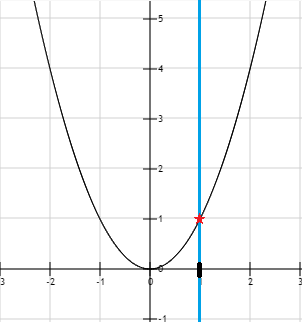With an equality constraint x=1, the minimum is 1

Looking at this example, you might feel like equality constraints are useless. This is not the case because most of the time optimization problems are performed in more than one dimension. So you could try to minimize a function $f(x,y)$ with only an equality constraint on $x$ for instance.

#### Inequality constraint

What if we now use an inequality constraint? It gives us a problem of the form:

This time, we can try more value of $x$. For instance, $x=2$ respects the constraint, so it could potentially be a solution to the problem. In the end, we find that the function $f$ has once again its minimum at $x=1$ under the constraint.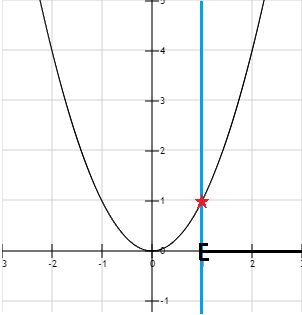Inequality constraint

On the graph above, the feasible region is shown in black bold it is a set of values of $x$ we are authorized to use. It is also called, the feasible set.

In mathematical notation we can write it:

$R$ is the set of values of $x$ for which the constraints are satisfied.

#### Combining constraints

It is possible to add several constraints to an optimization problem. Here is an example with two inequality constraints and its visual representation: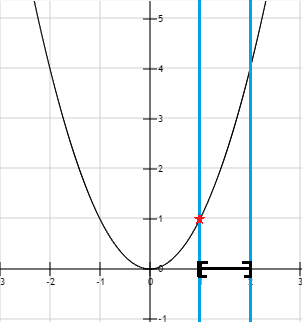Combining constraints restrict the feasible region

Note that we can also mix equality and inequality constraints together. The only restriction is that if we use contradictory constraints, we can end up with a problem which does not have a feasible set:

Indeed, for a constraint to be respected, it must be true. When there is several constraints, they all must be true.  In the example above, it is impossible for $x$ to equal 1 and to be less than zero at the same time.

For a function $f:\mathcal{X}\to\mathcal{Y}$ with one equality constraint $g(x)$ and one inequality constraint $h(x)$ the feasible set is :

### What is the solution of an optimization problem?

The optimal value $x^*$ of the optimization problem:

is:

Basically, it says in mathematical notation that the optimal value is the infimum of $f(x)$ with all the constraints respected.

## How do we find the solution to an optimization problem with constraints?

We will be using the Lagrange Multipliers. It is a method invented by the Italian mathematician, Joseph-Louis Lagrange around 1806.Joseph Louis Lagrange

### Lagrange multipliers

As often, we can find a pretty clear definition on Wikipedia:

In mathematical optimization, the method of Lagrange multipliers is a strategy for finding the local maxima and minima of a function subject to equality constraints. (Wikipedia)

The critical thing to note from this definition is that the method of Lagrange multipliers only works with equality constraints. So we can use it to solve some optimization problems: those having one or several equality constraints.

But don't worry, the Lagrange multipliers will be the basis used for solving problems with inequality constraints as well, so it is worth understanding this simpler case 🙂

Before explaining what the idea behind Lagrange multipliers is, let us refresh our memory about contour lines.

### Contour lines

It is important to understand contour lines to appreciate better Lagrange multipliers.

A contour plot is a way to visualize a three-dimensional function into two dimensions.

For instance, the picture below shows the function $x+y$ displayed in 3D (left) and with a contour plot (right):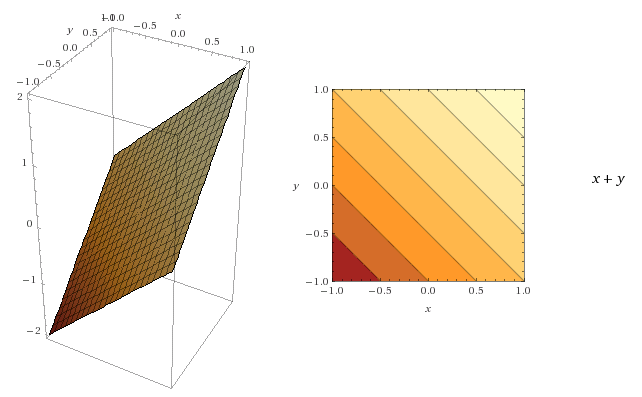Key concepts regarding contour lines:

• for each point on a line, the function returns the same value
• the darker the area is, the smallest the value of the function is

You can see the last point illustrated in the two figures below. Even if they have the same lines, the change of color allows us to draw a 3D picture of the function in our mind.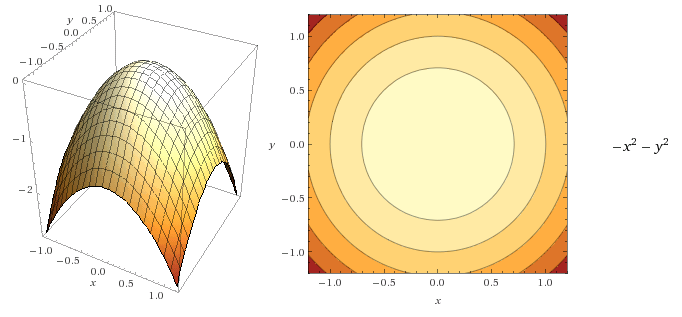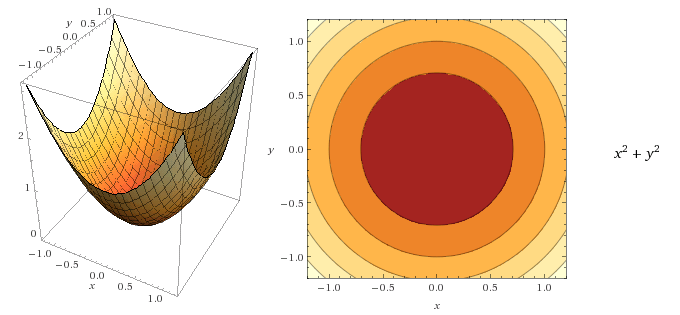Moreover, the gradient of a function can be visualized as a vector field, with the arrow pointing in the direction where the function is increasing: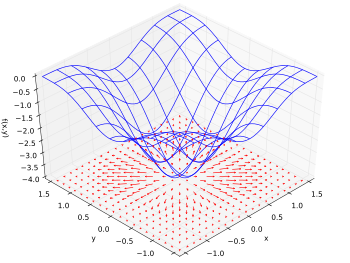The gradient of the function is projected as a vector field (Wikipedia)

It turns out we can easily draw gradient vectors on a contour plot:

• they are perpendicular to a contour line
• they should point in the direction where the function is increasing

Here is a representation on two contours plots: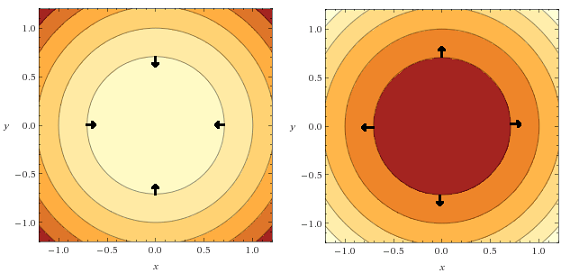### Back to Lagrangian multipliers

Let us consider the following optimization problem:

The objective function $f(x,y)$ and the constraint function $g(x,y)$ can be visualized as contour in the figure below: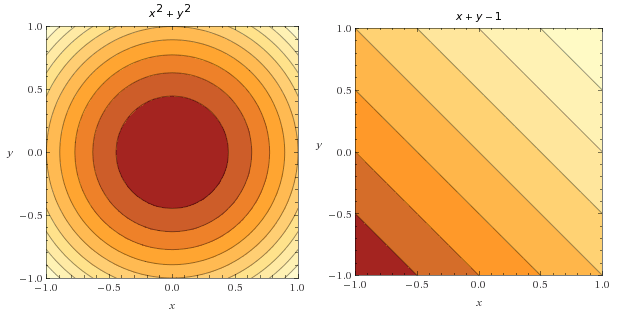It is interesting to note that we can combine both contour plot to visualize how the two functions behave on one plot. Below you can see the constraint function depicted by blue lines.
Also, I draw some gradient vectors of the objective function (in black) and some gradient vectors of the constraint function (in white).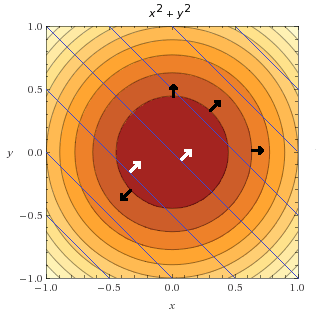However, we are not interested in the whole constraint function. We are only interested in points where the constraint is satisfied, i.e. $g(x,y)=0$.

It means that we want points where:

$x+y-1=0$

$x+y=1$

$y=1-x$

In the graph below we plot the line $y=1-x$ on top of the objective function. We saw earlier that this line is also called the feasible set.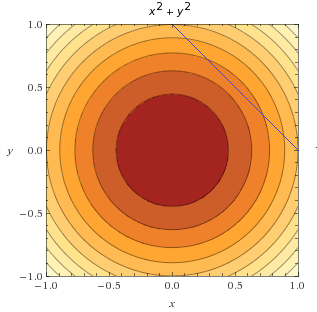What did Lagrange find? He found that the minimum of $f(x,y)$ under the constraint $g(x,y)=0$ is obtained when their gradients point in the same direction. Let us look at how we can find a similar conclusion.

In the figure below, we can see the point where the objective function and the feasible set are tangent. I also added some objective function gradient vectors in black and some constraint gradient vectors in white.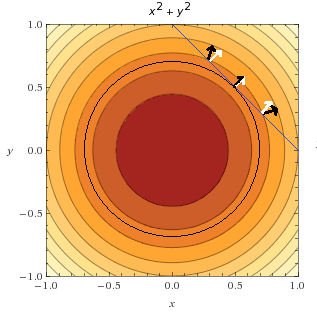We can see that the is only one point where two vectors point in the same direction: it is the minimum of the objective function, under the constraint.

Imagine you put your finger on the first point with arrows at the top of the figure. Here you evaluate the objective function, and you find out it returns some value $v$. Now, you wish to know if there is a smaller value. You must stay on the blue line, or the constraint will be violated. So you can either go to the left or the right.

You would like to go left, but then you see that the gradient of the objective function is pointing towards the left (remember it is always pointing towards greater values) so it might not be a good idea.  To be sure, you try and go to the left. You find out that the objective function returns $v+1$, so it is increasing which is not okay. Going to the right, this time, the objective function returns $v-1$, it is good you are going in the correct direction. So you continue like that until you reach the center point where the two arrows are parallel. But then, once you move a little bit you find out that the objective function is increasing again. As you can not move right or left anymore without increasing the objective function, you conclude this point is the minimum.

#### How does it translate mathematically?

Lagrange told us that to find the minimum of a constrained function, we need to look or points were $\nabla f(x,y) = \lambda \nabla g(x,y)$.

But what is $\lambda$ and where does it come from?

It is what we call a Lagrange multiplier. Indeed, even if the two gradient vectors point in the same direction, they might not have the same length, so there must be some factor $\lambda$ allowing to transform one in the other.

Note that this formula does not require the gradients to have the same direction (multiplying by a negative number will change the direction), but only to be parallel. It is because it can be used to find both maximum and minimum at the same time (see Example 1 of this article if you want to see how).

#### How do we find points for which $\nabla f(x,y) = \lambda \nabla g(x,y)$ ?

Note that,  $\nabla f(x,y) = \lambda \nabla g(x,y)$ is equivalent to:

$\nabla f(x,y) -\lambda \nabla g(x,y) = 0$

To make things a little easier, we notice that if we define a function:

$L(x,y,\lambda) = f(x,y)-\lambda g(x,y)$ then its gradient is:

$\nabla L(x,y,\lambda) = \nabla f(x,y)-\lambda \nabla g(x,y)$

This function $L$ is called the Lagrangian, and solving for the gradient of the Lagrangian (solving $\nabla L(x,y,\lambda) = 0$) means finding the points where the gradient of $f$ and $g$ are parallels.

### Let us solve this example using the Lagrange multiplier method!

Remember, the problem we wish to solve is:

#### Step 1: We introduce the Lagrangian function

$L(x,y,\lambda) = f(x,y)-\lambda g(x,y)$

$\nabla L(x,y,\lambda) = \nabla f(x,y)-\lambda \nabla g(x,y)$

#### Step 2: We solve for its gradient

We solve :

$\nabla L(x,y,\lambda) = 0$

which means solving the following system of equations:

$\begin{cases} \frac{\partial{L}}{\partial x} = 0\\ \frac{\partial{L}}{\partial y} = 0 \\ \frac{\partial{L}}{\partial \lambda} = 0 \end{cases}$

$\begin{cases} 2x-\lambda = 0\\ 2y-\lambda = 0 \\ -x-y+1= 0 \end{cases}$

By multiplying the second equation by -1 and adding the first and second equation we get:
$2x-2y=0$
so:
$x=y$

We replace $y$ by $x$ in the third equation:
$-x-x+1=0$
$-2x+1=0$
$-2x=-1$
$x=\frac{1}{2}$

We replace $x$ by $\frac{1}{2}$ in the first equation:
$2\frac{1}{2}-\lambda=0$
$1-\lambda=0$
$\lambda=1$

We have found a solution : $x=\frac{1}{2}$$y=\frac{1}{2}$ and $\lambda=1$

We conclude that the minimum of $f$ under the contraint $g(x,y)=0$ is obtained for $x=\frac{1}{2}$$y=\frac{1}{2}$.

Let us verify if it makes sense with our graphical analysis on the graph below: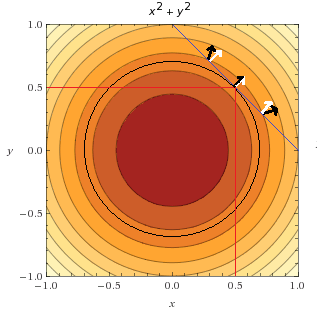The minimum of f is at x=1/2 and y=1/2

We can see that indeed the point $(x,y)=(\frac{1}{2},\frac{1}{2})$ is on the feasible set (the blue line) and it is the one we identified earlier.

## Conclusion

In this article, we learned an important concept in optimization: duality. Moreover, we discovered that an optimization problem can have equality and inequality constraints. Eventually, we learned what Lagrange multipliers are and how we can use them to solve an optimization problem with one equality constraint.

If you wish to learn more about Lagrange multipliers in the context of SVM, you can read this very good paper showing how to use them with more equality constraints and with inequality constraints.